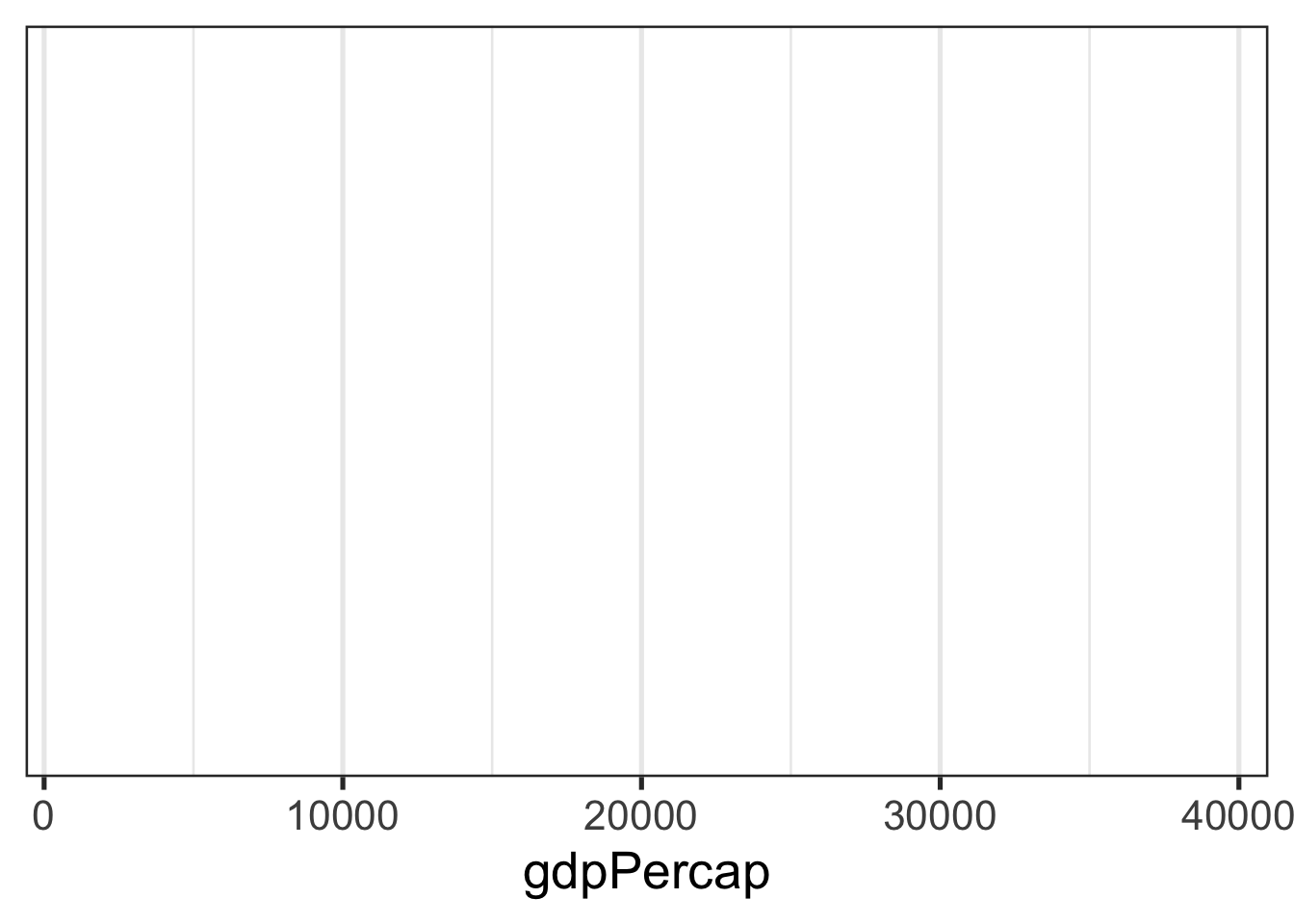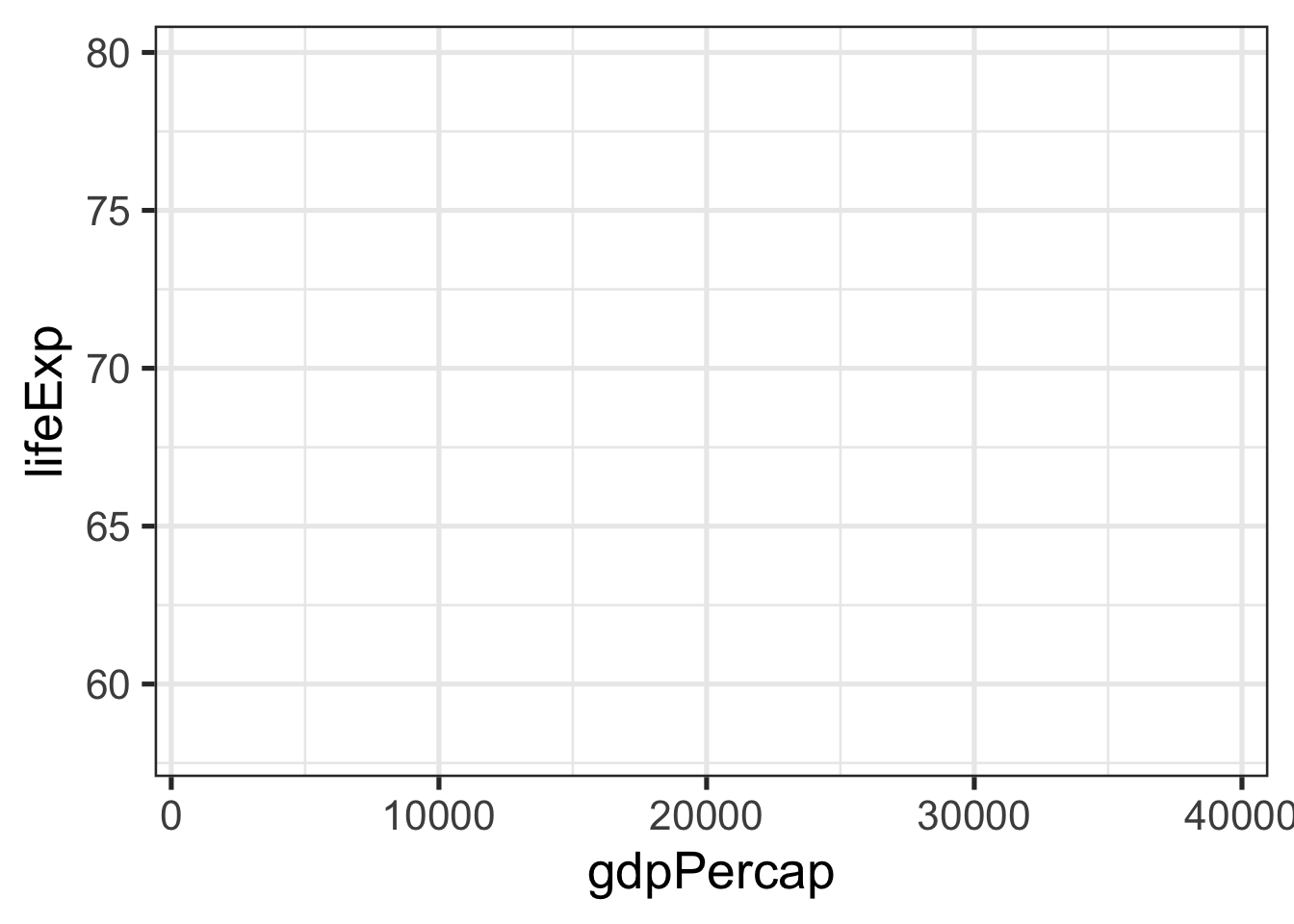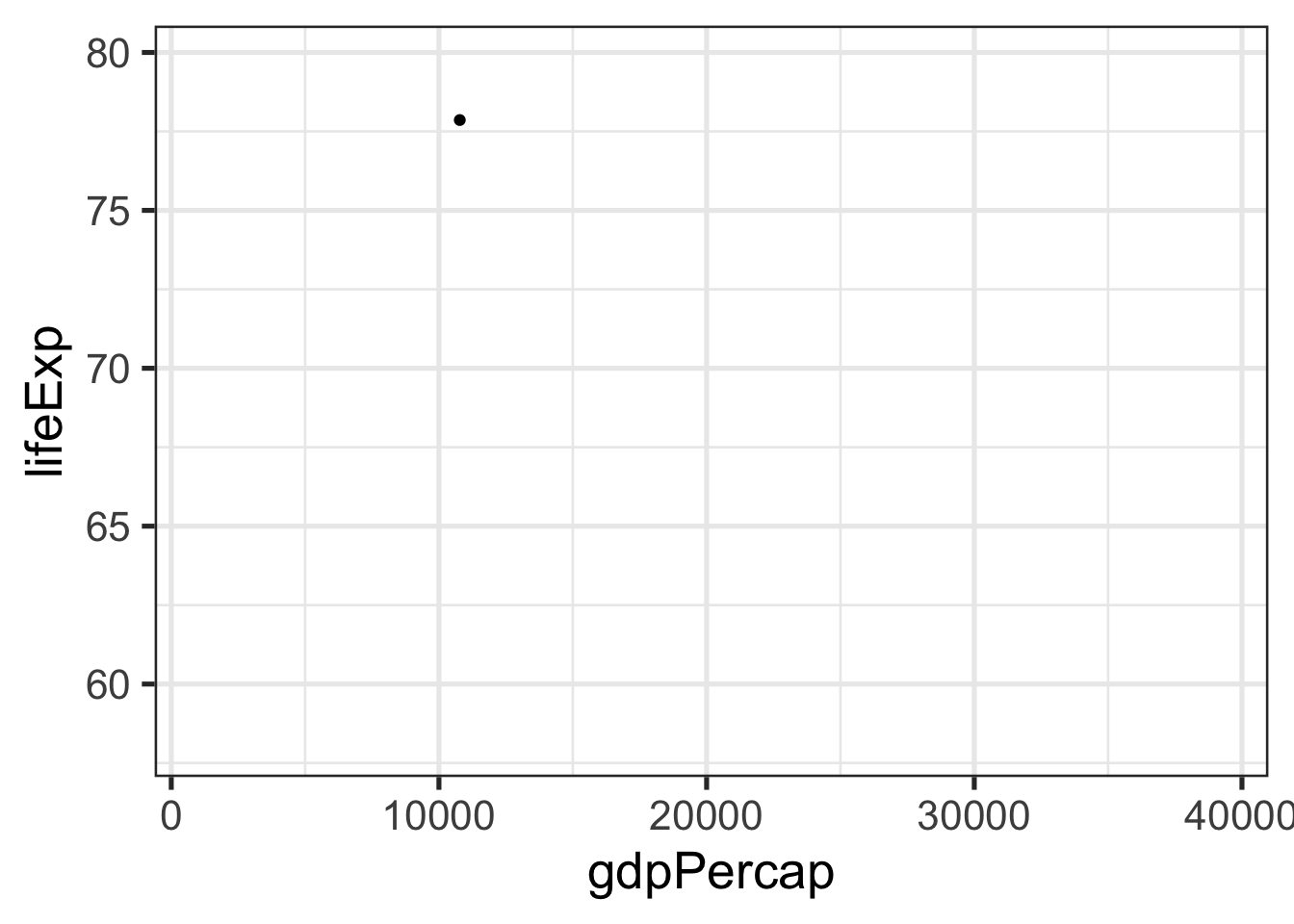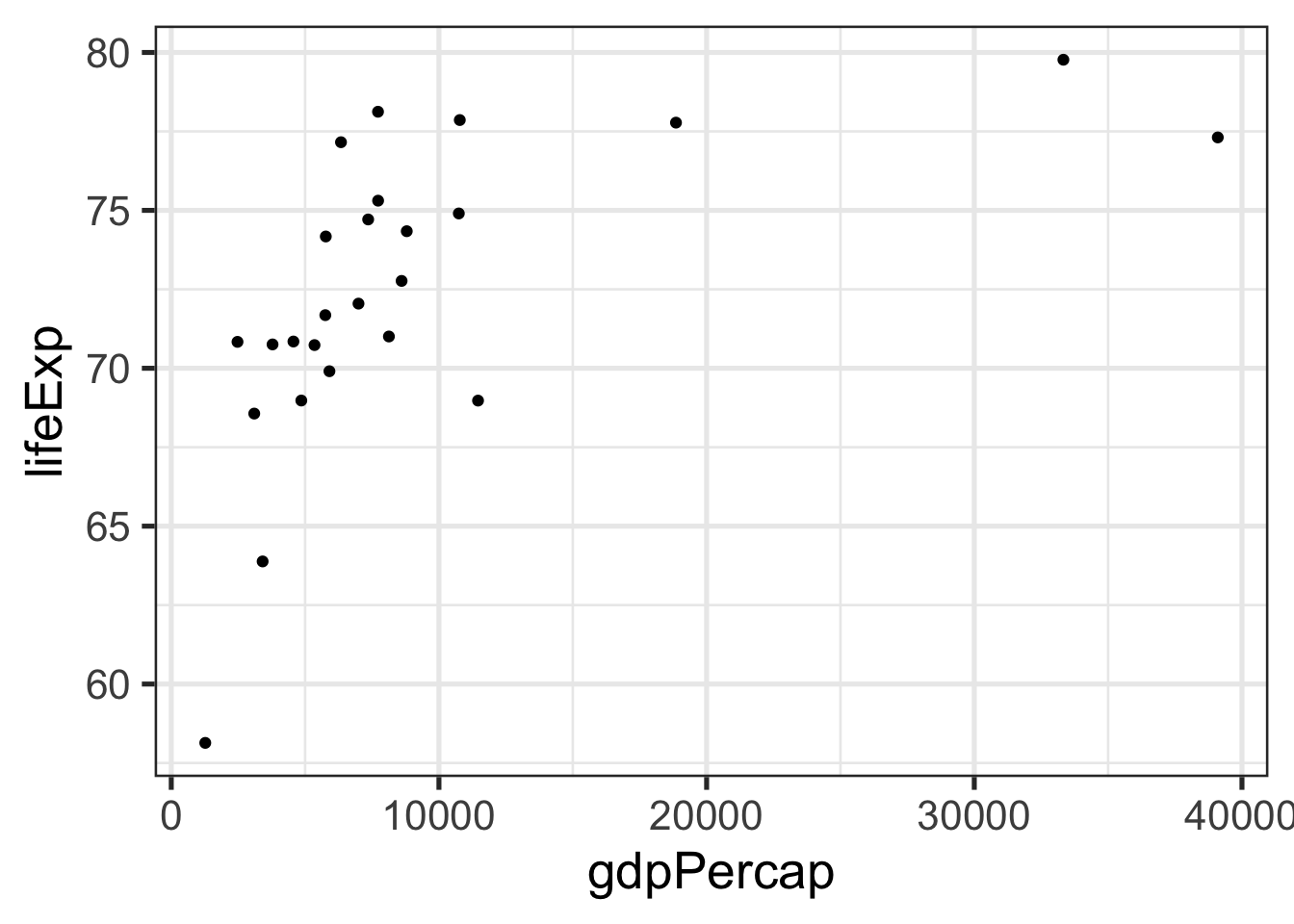# Layered Presentation of Graphics with +aes() in ggplot2

Here is the post is about how to implement a layered presentation of a graphics. Matthew Blackwell tweeted about the concept earlier this year, which seemed to reasonated with a lot of folks - to the tune of > 1500 likes! Practical, pedegogical stuff tends to get people excited. True for me too.

Anyway, this to me sounded a lot like the ggplot “layered grammar of graphics” ideology, and I retweeted this thought which resonated with a lot fewer people!

So then I set out to write an implementation with ggplot. I actually wrote a couple of implementations and what follows is my favorite, which is demonstrated using a year of data from the gapminder data package.

``````library(tidyverse)
library(gapminder)
data = gapminder %>% filter(continent == "Americas" & year == 2002)``````

# Step 1: Just x-axis (explain it)

``````ggplot(data) +              # declare the data you want to use
aes(x = gdpPercap) +      # declare the aesthetic mapping for x
theme_bw(base_size = 20)  # we want label sizes to be a bit bigger``````# Step 2: Just y-axis (explain it)

``````last_plot() +      # we just add to the last plot
aes(y = lifeExp) # add the aesthetic mapping for y``````# Step 3: Add 1 data point (explain it)

``````last_plot() +
geom_point() +  # declare the geom to add
aes(alpha = country == "Chile") +  # alpha is transparency aesthetic
scale_alpha_discrete(guide = FALSE, range = c(0,1)) ``````Above, we use an extreme range, so Chile is fully opaque and the rest of the points are fully transparent. So the point for Chile is the only point that will appear.

# Step 4: Plot the rest of the data (explain it)

Now we overwrite the alpha levels so that all are observations (countries) are represented with a fully opaque point.

``````last_plot() +
scale_alpha_discrete(guide = FALSE, range = c(1,1)) ``````Done!

So, a (big) bonus of this exercise was discovering that you can use aes() to set global aesthetics outside of the ggplot() function. This is not the usual way that ggplot is taught (see the RStudio Cheatsheats for example) but I really like it, because using it gives you even more of that “building-up-the-plot”, step-by-step, layered feel that makes ggplot so attractive!

##### Evangeline Reynolds
###### Visiting Teaching Assistant Professor

My research interests include international institutions, causal inference, data visualization, and computational social science and pedagogy.